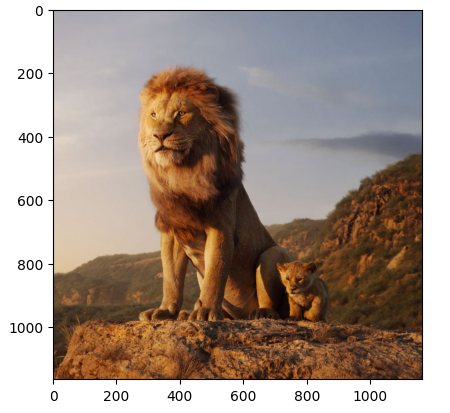# Utils.draw_bounding_boxes() outputs black image

Hi all,

I have just started tinkering with the PyTorch framework.
I am getting an error while using utils library to draw the bounding boxes.

Here is the code:

``````#draw the bounding boxes around the objects detected in the image
try:
input_value = img_tensor.cpu() #copying image transformed tensor data from GPU
plt.imshow(utils.make_grid(input_value, nrow=1).permute(1,2,0)) #display the input image
plt.show()
boxes_tensor = torch.Tensor(pred_boxes) #converting boxes into a tensor
preprocess_img = transforms.Compose([transforms.ToTensor()])(cpy_img) #transforming image to a tensor from the same input file
out_img_tensor = preprocess_img.squeeze(0)
out_img_type = out_img_tensor.type(torch.uint8) #converting the flaot tensor into uint8 as required by utils
final_output = utils.draw_bounding_boxes(out_img_type, boxes_tensor, pred_classes) #draw the bounding boxes
plt.imshow(utils.make_grid(final_output, nrow=1).permute(1,2,0)) #display the ouput result
plt.show()
except Exception as er4:
print(er4)
``````

Please find the below images for reference:Thank you

The input image was:I guess the transformation to `uint8` could create an all-zero image as described in the comments:

``````# ToTensor returns a tensor in the range [0, 1]
preprocess_img = transforms.Compose([transforms.ToTensor()])(cpy_img)
out_img_tensor = preprocess_img.squeeze(0)
# transform float32 [0, 1] to uint8 [0, 255], which will create the black image
out_img_type = out_img_tensor.type(torch.uint8)
``````

so you could scale the pixel values to e.g. `[0, 255]` before transforming them.

Hi @ptrblck , I have tried couple of approaches after your comments to tranform the pixel value but, I haven’t landed in a correct solution. However, I have tried few approaches such as converting the tensor into a numpy array → multiplying the array by 255 and again converting back to tensor. still, I haven’t found a good the output. If you can point me towards a good article/tutorial that would be helpful. Thanks

Multiplying by `255` works for me, so I’m unsure which approaches you’ve used:

``````# create random image
cpy_img = np.random.randint(0, 255, (100, 100, 3)).astype(np.uint8)
cpy_img = PIL.Image.fromarray(cpy_img)

# ToTensor returns a tensor in the range [0, 1]
preprocess_img = transforms.Compose([transforms.ToTensor()])(cpy_img)
out_img_tensor = preprocess_img.squeeze(0)
# transform float32 [0, 1] to uint8 [0, 255], which will create the black image
out_img_type = out_img_tensor.type(torch.uint8)
print((out_img_type==0).sum()) # all zeros
plt.imshow(out_img_type.permute(1, 2, 0).numpy()) # all black

print(out_img_tensor.min(), out_img_tensor.max())
> tensor(0.) tensor(0.9961)

out_img_tensor = out_img_tensor * 255
out_img_type = out_img_tensor.type(torch.uint8)
plt.imshow(out_img_type.permute(1, 2, 0).numpy()) # works
``````

I can’t point you to a specific article, as the error is caused by transforming floating point data in the range `[0, 1]` to `uint8`, which will create an (almost) all-black image.

1 Like

Thank you @ptrblck

I got the desired output.1 Like